# Inter Maths Solutions for Applications of Derivatives exercise 10(a)

Intermediate Mathematics 1B Exercise 10(a) Applications of Derivatives textbook Solutions are given.

Study the textbook Inter maths 1b Applications of Derivatives very well.

Observe the example problems and solutions given in the textbook.

Observe the given below solutions and try them in your own method.

You can also see the solutions

SSC Maths text book Solutions class 10

Inter maths 1A textbook solutions

Inter Maths 1B textbook solutions

Inter Maths 1IA text book solutions

Inter Maths IIB text book solutions

Applications of Derivatives

Exercise 10(a)

Exercise 10(b)

Exercise 10(c)

Exercise 10(d)

Exercise 10(e)

Exercise 10(f)

Exercise 10(g)

Exercise 10(h)

M

## Solutions for exercise 10(a) Applications of Derivatives Inter Maths 1b

Chapter – 10

Applications of Derivatives

Exetcise 10(a)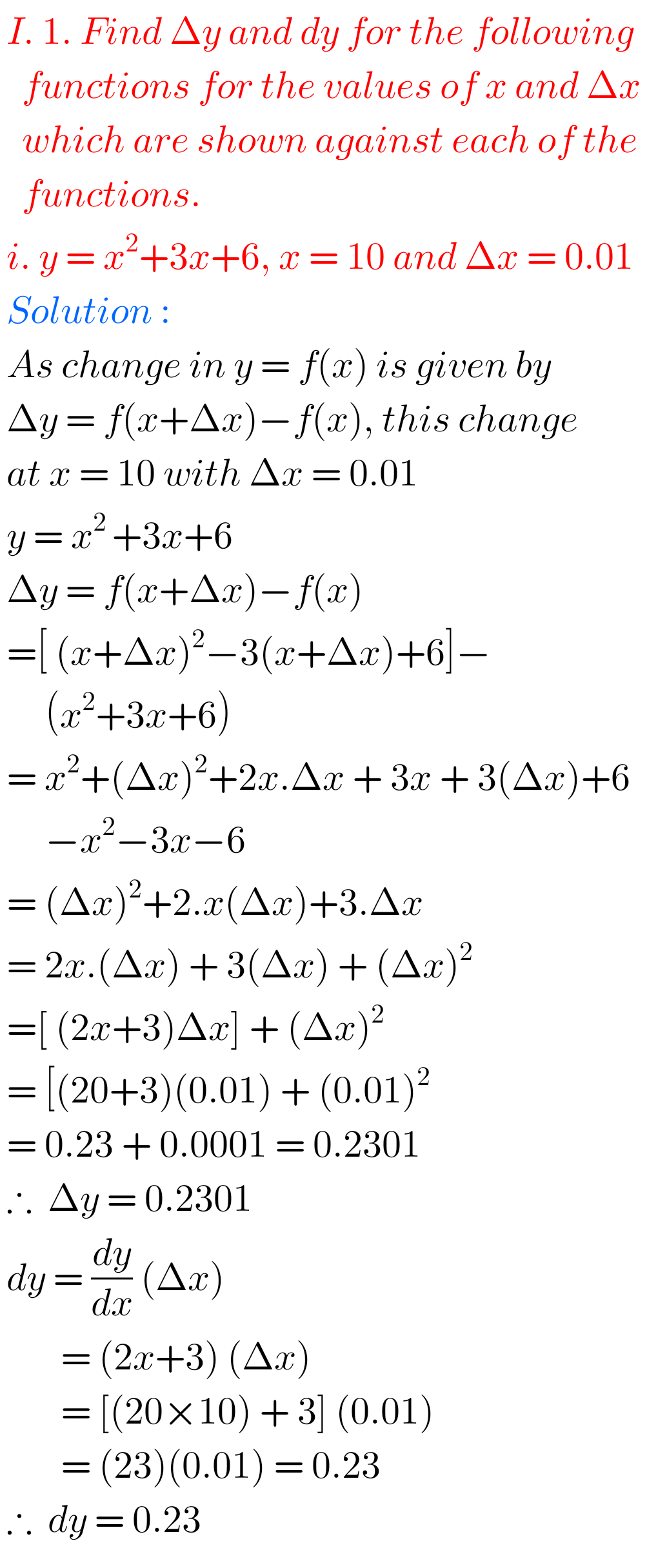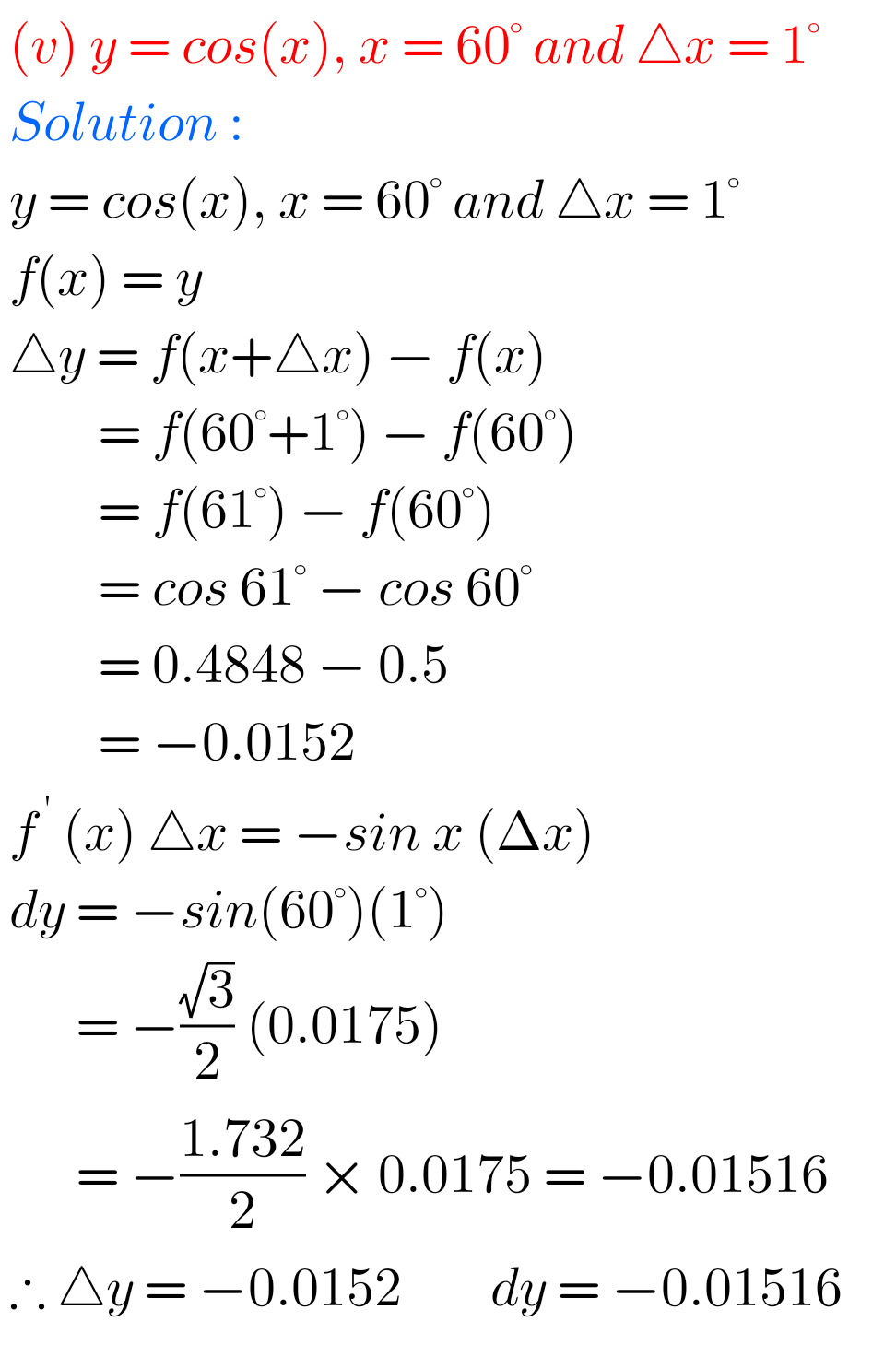## Exercise 10(a) Solutions Applications of Derivatives Inter maths 1b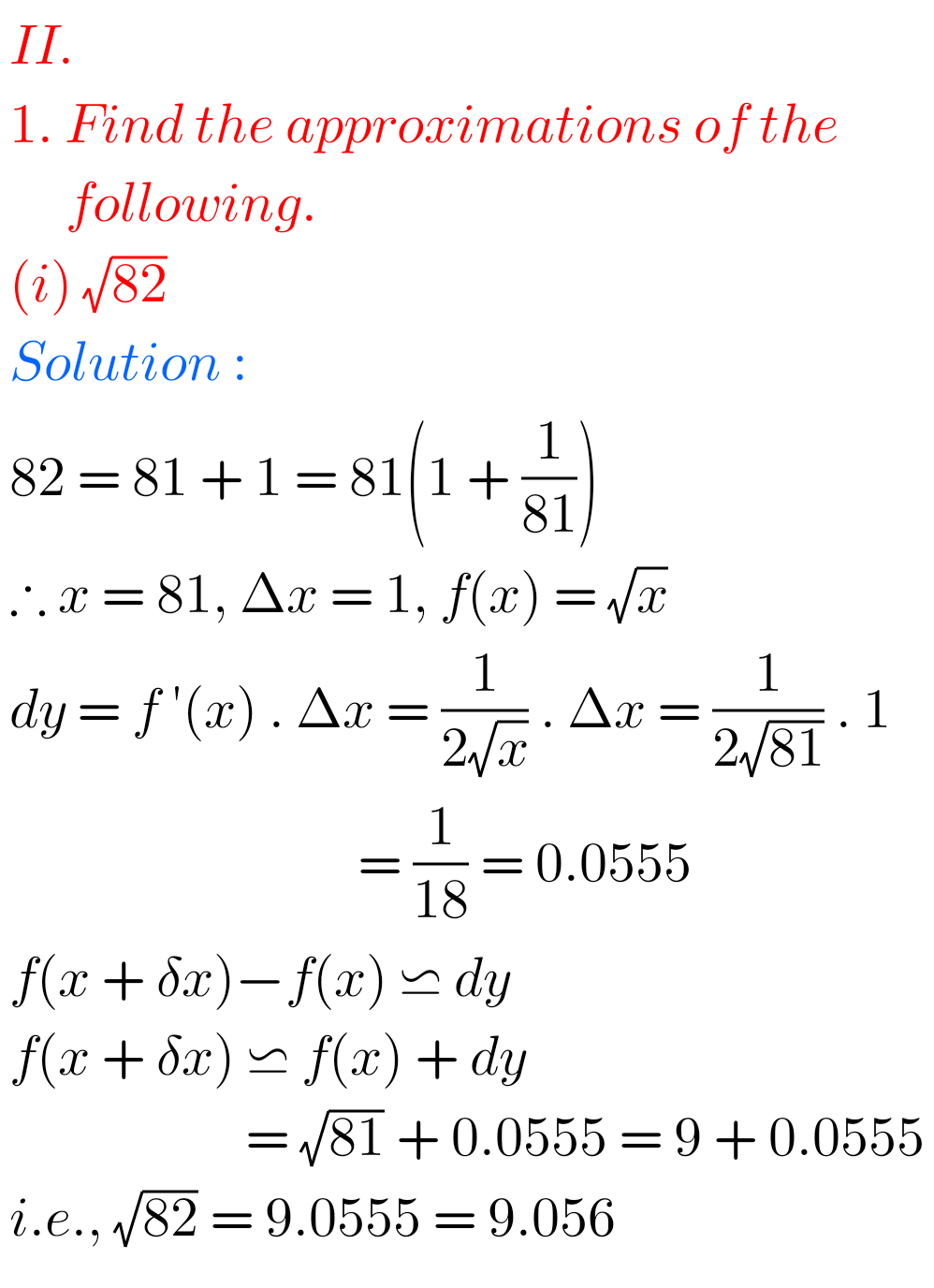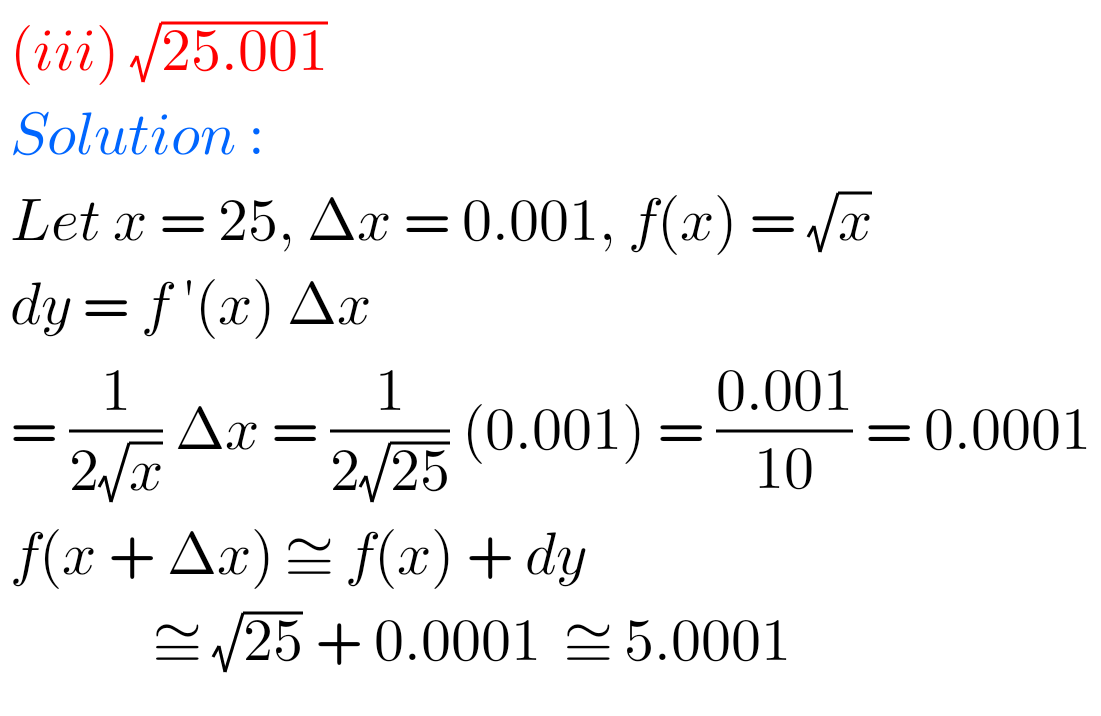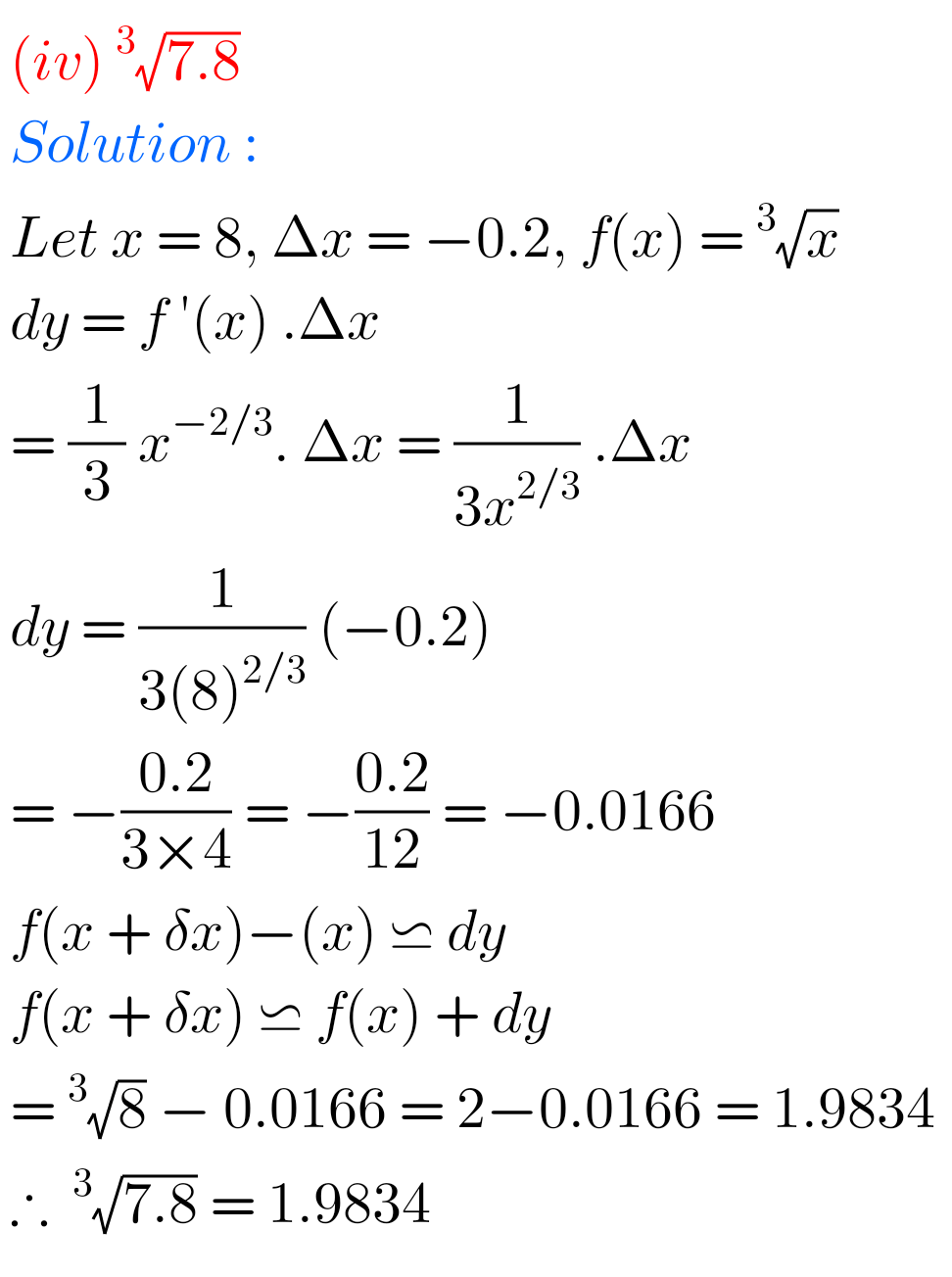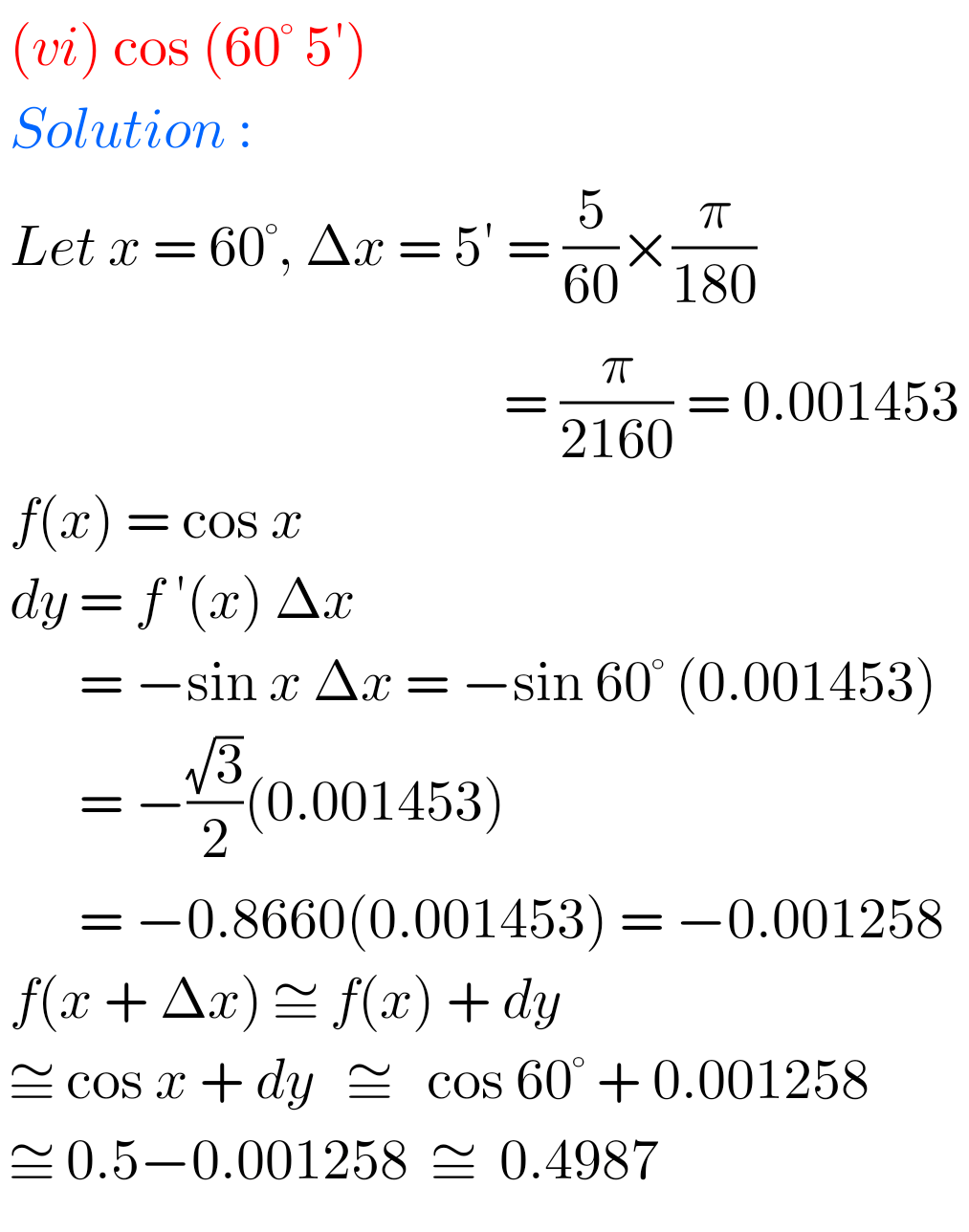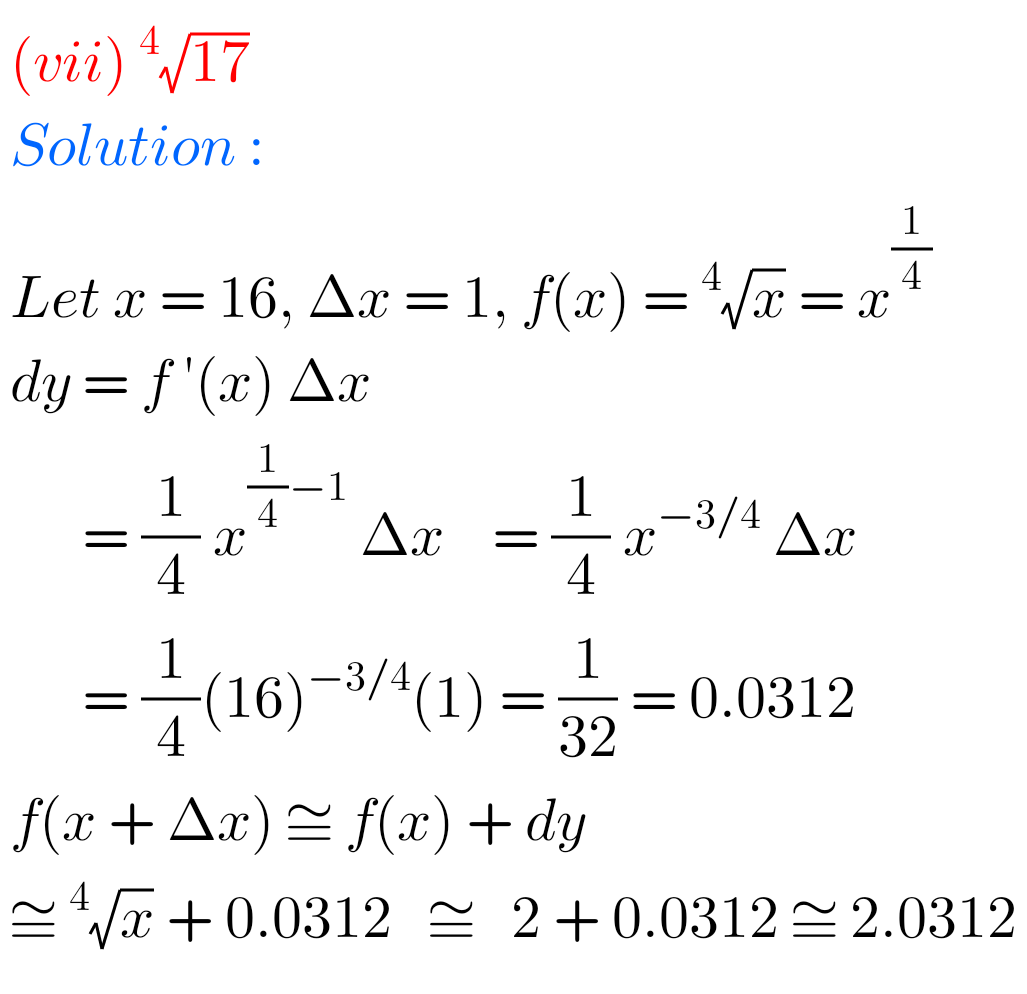## Maths Inter 1b Applications of Derivatives exercise 10(a) solutions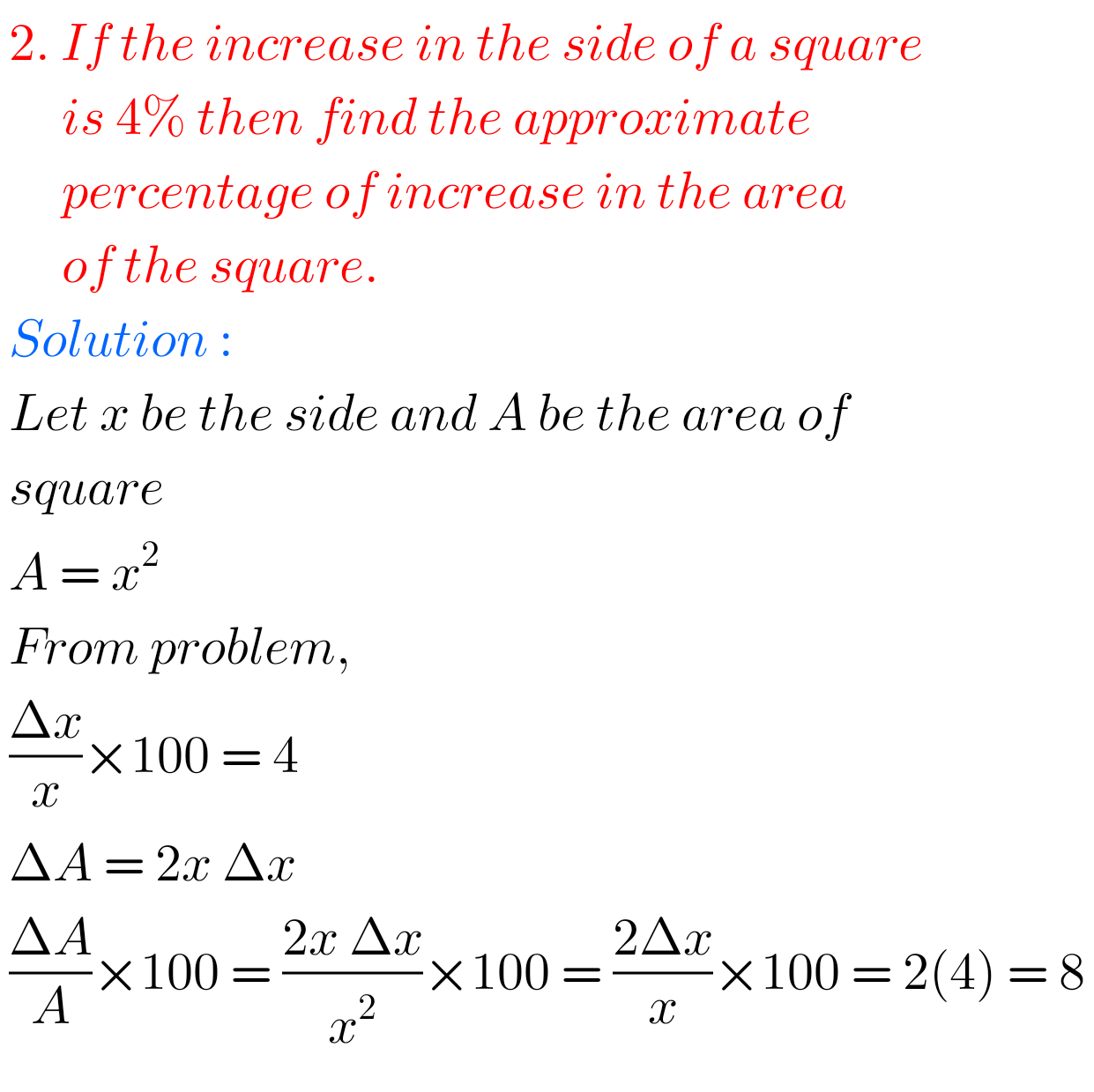Note : Observe the solutions and try them in your own method.Nios solutions for maths 311 Sets 1.1 book 1

Nios Solutions for maths 311 book Sets 1.2